DraftKings 2019 Big 12 Odds
-670
0.15 to 1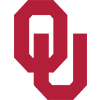Oklahoma
73.7% implied probability

+400
4 to 1Baylor
17.0% implied probability

+2000
20 to 1Texas
4.0% implied probability

+5000
50 to 1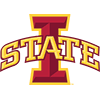Iowa State
1.7% implied probability

+5000
50 to 1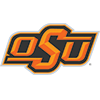Oklahoma State
1.7% implied probability

+6600
66 to 1Kansas State
1.3% implied probability

+50000
500 to 1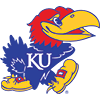Kansas
0.2% implied probability

+50000
500 to 1West Virginia
0.2% implied probability

+50000
500 to 1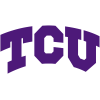TCU
0.2% implied probability

+50000
500 to 1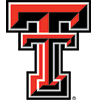Texas Tech
0.2% implied probability